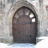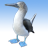# Boundary Effects in Average Nearest Neighbor

2782
6
04-25-2012 08:25 PMNew Contributor III
Hello Everyone,

I am working on recreating the Average Nearest Neighbor tool and was wondering if anybody knows A) does the NN tool in ArcToolbox take into account edge/boundary effects and B) how does the script do this (what method does it use)?  Any help in this would be great.  So far to account for edge effects in my own work I have created an inner buffer zone for my points and removed all nearest neighbor distances greater than e^(natural log(mean + (2 * standard deviations))).  Also, if anybody has any pointers on what other edge-effect corrections I could use.

Thank you all,

Winn
Tags (2)
6 RepliesOccasional Contributor III
It looks like you are implementing the "Guard" subregion correction. Another option is where you assume a "minimum distance" neighbor to the boundary observations and incorporate these values into the NNI. Other options are the "Donnelly" rectangular correction and the "Kaplan-Meier" correction that uses a cumulative distribution function.

I would pose the question: if you are going to the trouble of "re-creating" the NNI statistic (I assume in Python) then why are you not implementing a more modern counterpart (i.e., K, G-hat, F-hat) that accounts for the know limitations of the Clarke-Evans NNI? ESRI has developed methods for linking R and ArcGIS (through Python) that could be leveraged to provide a suite of well developed PPA statistics.

I would recommend the following readings in point pattern statistics and the NNI:

Baddeley, A.J. and Gill, R.D. (1997) Kaplan-Meier estimators of interpoint distance distributions for spatial point processes. Annals of Statistics 25:263-292.

Cressie, N.A.C. (1991) Statistics for spatial data. John Wiley and Sons, 1991.

Diggle, P.J. (1983) Statistical analysis of spatial point patterns. Academic Press.

Donnelly, K. (1978) Simulations to determine the variance and edge-effect of total nearest neighbour distance. In Simulation methods in archaeology, Cambridge University Press, pp 91�??95.

Hanisch, K.-H. (1984) Some remarks on estimators of the distribution function of nearest-neighbour distance in stationary spatial point patterns. Statistics 15:409�??412.

Ripley, B.D. (1988) Statistical inference for spatial processes. Cambridge University Press.New Contributor III
Jeff,

Thank you for the response, I will look into the other methods and articles you provided.  Let me also clarify what I am really trying to accomplish.  As part of my MS thesis, I wrote a python script and built a tool that will calculate a 2D and 3D NNI value for 2- and 3-dimensional features for some paleontological data I was given here at ETSU (using the 3-dimensional equation provided by Clarke and Evans (1979)).  What I am interested in doing is incorporating a boundary correction method into the script and automating that process.  What I found with the Guard correction method is that I could at least manually sort through the points and pull out any NN distances for both dimensions that are greater than the resulting value of the natural log equation I used.  With current technologic advancements and tools in ArcGIS, R, etc., would it be possible to implement the K, G-hat, or other boundary corrections for 3D feature sets into a python script?

WinnOccasional Contributor III
Winn,
I am unclear on exactly what you mean by 3D point patterns. Do you mean "marked point process" (a point process with an associate value at each sample location)? There are a variety of marked point process statistics available in the R libraries: "spatstat", "spdep", "PtProcess", and "MarkedPointProcess"  among others. Please be mindful of testing for the model assumptions of "homogenous spatial process". If your point process is inhomogenous the resulting statistic is invalid because the assumed null of CSR (Complete Spatial Randomness) following a Poisson process is incorrect. Inhomogenous spatial processes follow a conditional intensity function and not CSR. Some common test statistics available in spatstat are Kernel Smoothing, empty space and pair correlation functions. The Kernel Smoothing method is quite intuitive, just look for a consistent trend in the resulting plot. If you have localized areas of differing intensity then you have an inhomogenous spatial process. I would highly recommend exploring the K function. There are both homogenous and inhomogenous versions of the statistic available. Alternatively you may want to consider fitting a spatial process or Gibbs model so you can explicitly account for an intensity function and explore specific covariates.

I am not much into Python but you may want to look into the Python spatial library PySAL. I have no idea if there are any point process statistics but, given the developers behind the effort, I am fairly confident that they have some robust spatial weighting matrix functions available. This may be a good jumping-off point for developing your own functions in Python.

PySAL Homepage
https://geodacenter.asu.edu/pysalNew Contributor III
Jeff,

What I mean by "3D point patterns" is that each point has lat, lon, and elevation values and are represented by a 3D feature.  In essence the tool I created calculates the NNI for nearest neighbor distances for the 2nd and 3rd dimension, i.e. a nearest neighbor distance is determined using Euclidean measurements just as in ESRI's Average Nearest Neighbor statistic.  What it lacks is an ability to define a study area boundary on the fly and to account for boundary effects.  What I would like to do is A) be able to define a 3D boundary using the points in the analysis (as when you don't provide a pre-defined boundary with ESRI's tool) and B) use that boundary to control for boundary effects.

WinnOccasional Contributor III
The spatstat (http://www.spatstat.org/spatstat/) library in R allows you to specify a boundary. The "owin" object class is what you pass to a given PPA model in spatstat to define the boundary. An owin object can be based either on a predefined boundary or a convex function. You can use the rgdal or maptools package to read (point or polygon) shapefiles. I understand that it is possible to compile GDAL with the ESRI API for FileGeodatabases but I have not tried it. If you read in a shapefile then you need to coerce the data from an sp to a spatstat owin or ppp object class.

As far as coding this in Python I would look at the nice example that ESRI provided on calling R from ArcToolbox.
http://resources.arcgis.com/gallery/file/geoprocessing/details?entryID=F855D6D1-1422-2418-A0B2-643E6...

This framework would allow to stay in the ArcGIS environment while calling R through a simple Python wrapper via ArcToolbox. There is really no need to reinvent the wheel here. The statistics, boundary specification and corrections, and simulation capacity that you want/need already exists in spatstat. This is a very mature and well respected library that is one of the de facto standards in spatial statistics. Here is a Journal of Statistical Software paper that introduces the library (http://www.jstatsoft.org/v12/i06).New Contributor III
This is great.  Thank you so much for helping me out with this, I will definitely check out the spastat library.